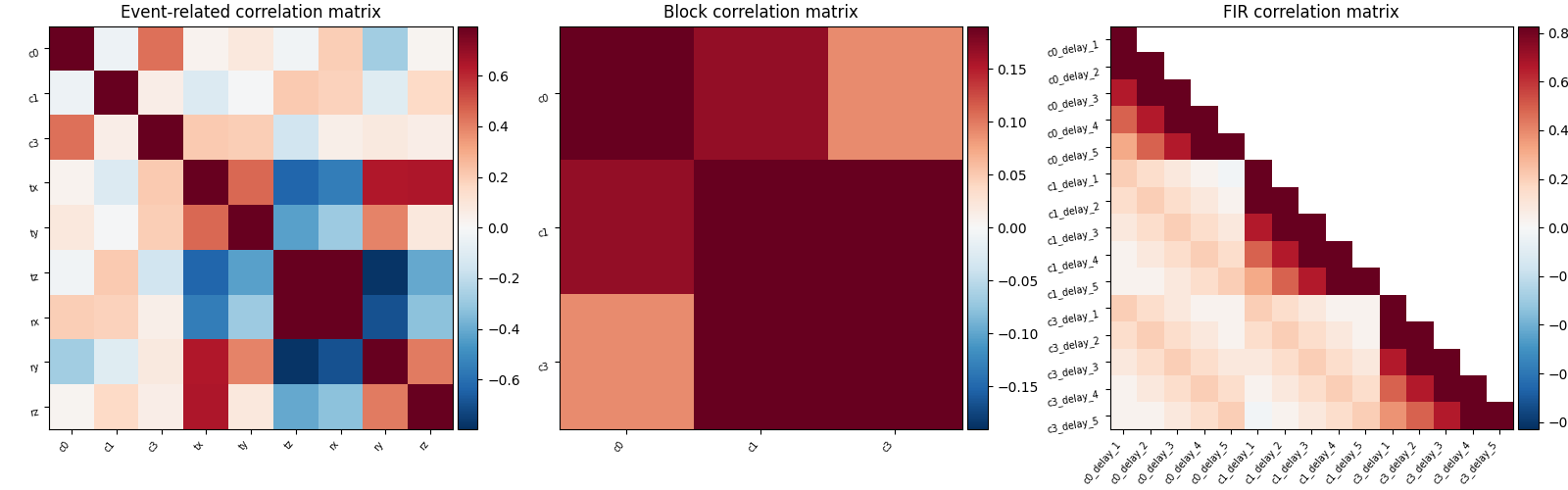# Examples of design matrices#

Three examples of design matrices specification and computation for first-level fMRI data analysis (event-related design, block design, FIR design).

This examples requires matplotlib.

```try:
import matplotlib.pyplot as plt
except ImportError:
raise RuntimeError("This script needs the matplotlib library")
```

## Define parameters#

At first, we define parameters related to the images acquisition.

```import numpy as np

tr = 1.0  # repetition time is 1 second
n_scans = 128  # the acquisition comprises 128 scans
frame_times = np.arange(n_scans) * tr  # here are the corresponding frame times
```

Then we define parameters related to the experimental design.

```# these are the types of the different trials
conditions = ["c0", "c0", "c0", "c1", "c1", "c1", "c3", "c3", "c3"]
duration = [1.0, 1.0, 1.0, 1.0, 1.0, 1.0, 1.0, 1.0, 1.0]
# these are the corresponding onset times
onsets = [30.0, 70.0, 100.0, 10.0, 30.0, 90.0, 30.0, 40.0, 60.0]
# Next, we simulate 6 motion parameters jointly observed with fMRI acquisitions
motion = np.cumsum(np.random.randn(n_scans, 6), 0)
# The 6 parameters correspond to three translations and three
# rotations describing rigid body motion
add_reg_names = ["tx", "ty", "tz", "rx", "ry", "rz"]
```

## Create design matrices#

The same parameters allow us to obtain a variety of design matrices. We first create an events object.

```import pandas as pd

events = pd.DataFrame(
{"trial_type": conditions, "onset": onsets, "duration": duration}
)
```

We sample the events into a design matrix, also including additional regressors.

```from nilearn.glm.first_level import make_first_level_design_matrix

hrf_model = "glover"
X1 = make_first_level_design_matrix(
frame_times,
events,
drift_model="polynomial",
drift_order=3,
hrf_model=hrf_model,
)
```

Now we compute a block design matrix. We add duration to create the blocks. For this we first define an event structure that includes the duration parameter.

```duration = 7.0 * np.ones(len(conditions))
events = pd.DataFrame(
{"trial_type": conditions, "onset": onsets, "duration": duration}
)
```

Then we sample the design matrix.

```X2 = make_first_level_design_matrix(
frame_times,
events,
drift_model="polynomial",
drift_order=3,
hrf_model=hrf_model,
)
```

Finally we compute a FIR model

```events = pd.DataFrame(
{"trial_type": conditions, "onset": onsets, "duration": duration}
)
hrf_model = "FIR"
X3 = make_first_level_design_matrix(
frame_times,
events,
hrf_model="fir",
drift_model="polynomial",
drift_order=3,
fir_delays=np.arange(1, 6),
)
```

Here are the three designs side by side.

```from nilearn.plotting import plot_design_matrix

fig, (ax1, ax2, ax3) = plt.subplots(figsize=(10, 6), nrows=1, ncols=3)
plot_design_matrix(X1, ax=ax1)
ax1.set_title("Event-related design matrix", fontsize=12)
plot_design_matrix(X2, ax=ax2)
ax2.set_title("Block design matrix", fontsize=12)
plot_design_matrix(X3, ax=ax3)
ax3.set_title("FIR design matrix", fontsize=12)
``````Text(0.5, 1.1753015208063937, 'FIR design matrix')
```

Let’s improve the layout and show the result.

```plt.subplots_adjust(left=0.08, top=0.9, bottom=0.21, right=0.96, wspace=0.3)
plt.show()
```Total running time of the script: ( 0 minutes 5.522 seconds)

Estimated memory usage: 9 MB

Gallery generated by Sphinx-Gallery